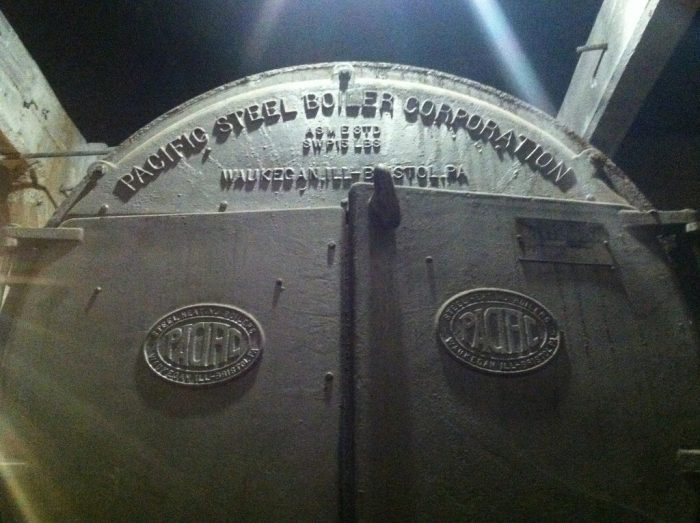## Sensible Heat in Air and WaterHere is another great explanation from Michael Housh from Housh Home Energy in Ohio.Thanks Michael!

I’m going to layout and compare the Sensible Heat Rate equations for both the air-side and water-side of HVAC, to help draw similarities and dive deeper into the science behind these equations.  This is the beginning of a series to try and help us all gain a deeper knowledge of where these equations come from. The more we learn about the two, the more similarities can be drawn between them. This will hopefully allow a technician to be more comfortable when faced with different systems in the field.  I should also note that while the equations can be complex, they are a great reference for those who would like to build them into spreadsheets (or other formats).

Sensible Heat Rate Equations:

 Air Water Q = 1.08 * CFM * T Q = 500 * GPM * T Where: Where: Q = sensible heat transferred (Btu/hr) Q = sensible heat transferred (Btu/hr) CFM = quantity of air (ft3/min) GPM = quantity of water (gallons/min) T= dry bulb temperature difference (°F) T= dry bulb temperature difference (°F)

The only thing I will say about the Delta-T side is that it is the measurement of dry-bulb temperature, this is something I think all technicians know and have a decent grasp on.

Like most things in our industry these are “rules of thumb” equations, however, both derive from the same lower level equation, which is as follows:

Q = M * C * T

Where:

Q = sensible heat transferred (Btu/hr)

M = mass of the fluid (lb/ft3)

C = specific heat of the fluid (Btu/lb)

T= dry bulb temperature difference (°F)

I’ve often heard Bryan say that air-conditioning is about moving pounds of refrigerant.   We move pounds of refrigerant to create air-conditioning, and we move pounds of air to deliver that air-conditioning to the space.  As you may have gathered from the above equation the Sensible Heat Rate is derived from moving pounds of a substance (in our case air or water).

I’m not going to dive into the details of the above equation at this time, but wanted to share where both of these equations stem from. What I’d like to breakdown in this article is a deeper understanding of where the 1.08 (air) and 500 (water) constants come from.   Both CFM and GPM are actually what provides the “pounds” of the fluid (air is a fluid), based on density and specific heat.

Density is defined as its mass per unit of volume (or weight per unit of volume), and specific heat is the rate at which an object will give off or absorb thermal energy.  Both density and specific heat are moving targets, but in the “rule of thumb” below are the values that are used.

 Density (lb/ft3) Specific Heat (Btu/lb) Air @ 70°F & at sea-level (14.7 psia) 0.075 0.24 Water @ 60°F 62.37 1.0

I’m going to solve for the water-side first.  We have to take into account that our measurement for water is Gallons Per Minute, so for anyone who doesn’t know, there are approximately 7.48 gallons in 1 cubic foot.  Using the density from the table above we can solve for the weight of one gallon of water 62.37 / 7.48 = 8.34 lb @ 60°F.  Since our end result of the Sensible Heat Rate equation is Btu/hour we have to convert our GPM -> GPH (gallons per hour).  So, our 500 is a simplification of the following values:

Constant = 8.34 (lbs/gal) * 60 (min) * 1 (specific heat) = 500

When we provide the GPM in the Sensible Heat Rate equation for water, we have already accounted for its density (mass), specific heat, and converted to gallons per hour.

Next, let’s look at the air-side.  Here we have to account (just like in the water-side), that our measurement is in Cubic Feet per Minute, and since we are solving for Btu/hour we will have to convert CFM – > CFH (cubic feet per hour), we also have to use the density to account for the mass of air that we are moving, and the specific heat.  So, our 1.08 is a simplification of the following values:

Constant = .075 (density [ lbs/ft3]) * 60 (min) * 0.24 (specific heat) = 1.08

So, just like the water side, when we provide CFM to the Sensible Heat Rate equation, we have already accounted for its density (mass), specific heat, and converted to cubic feet per hour.

I hope I haven’t utterly confused you on such a technical topic, but stay tuned for more in the series to help bring the Sensible Heat Rate equation (and the air / water side) closer together.

— Michael Housh

This site uses Akismet to reduce spam. Learn how your comment data is processed.

Scroll to top
Translate »

### Daily Tech Tip

Get the (near) daily Tech Tip email right in your inbox!